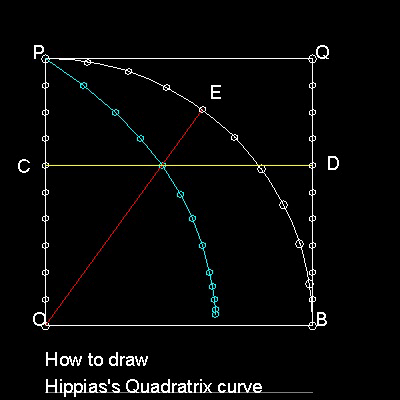Special Curves
Go to   Fun_Math Content Table   Trisecting an Angle   Special Curves

### Trisection using Special Curves

Hippias's(about 460BC-about 400BC) Trisection method is shown in the figure shown below.The procedure for Trisection is:

Step 1:Draw the quadratrix , then select a point "A" on this curve to define angle AOB

Step 2:Draw a line from "A" parallel to AB and the intersection with line PO is point "C".

Step 3:Find a point "D" on line CO such that OD = CO/3

Step 4:Draw a line from "D" parallet to OB, and find a point "E" ,intersecting this curve.

Angle EOB trisects angle AOB.

You can see the process in animation.

To create this drawing and animation:
Then from command line, type quadratrix_3

#### How to Draw a QuadratrixOBQP is a square.

Divide both OP and BQ in equal parts N.

Divide a quarter circle BP into the same number N. Point C,D & E arer such points.

Horizontal line CD(yellow) and polar line OE(red) intersect .

The locus of such points(cyan color) is the "Quadratrix".

You can see the process in animation.

To create this drawing and animation:
Then from command line, type quadratrix_2 for drawing quadratrix for 1000 division.
test_1  & test_2   for drawing manually.

If OP & OB are x & y axis respectively, the curve is expressed as y = x tan(p*y/2)

Using identity tan(a) = sin(a)/cos(a), and replacing (p/2)y = h

x = (2/p)*cos(h)*(h/sin(h))

As h approaches zero, both cos(h) and (h/sin(h)) approach 1.

So the x-coordinate of the point R,where the Quadratrix intersect X-axis is (2/p).

This means that length OR is used to get p value, and we now have succeeded in "Squaring the Circle". This is the reason why this curve is named "Quadratrix", i.e. curve for circle quadrature.

Go to   Fun_Math Content Table   Trisecting an Angle   Special Curves

All questions/suggestions should be sent to Takaya Iwamoto

Last Updated Nov 22, 2006